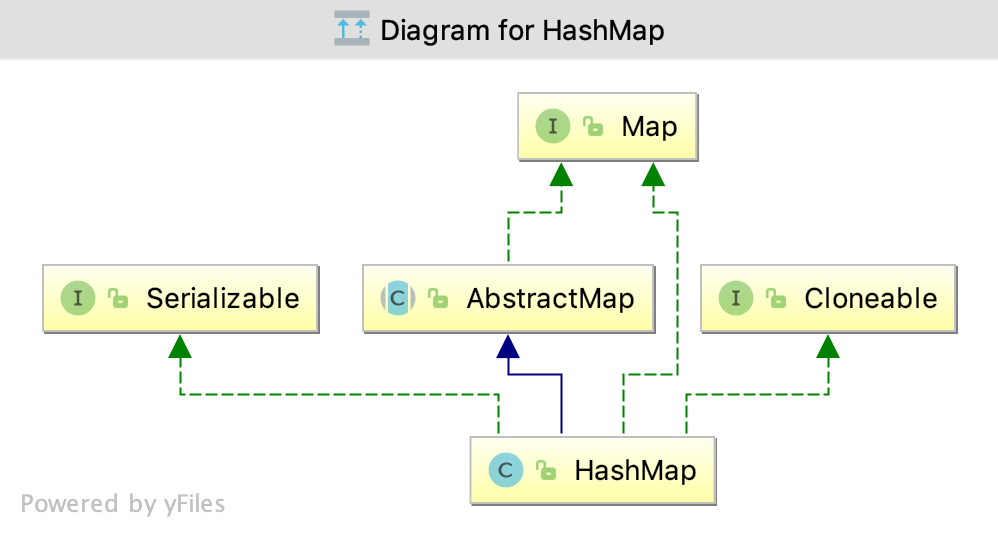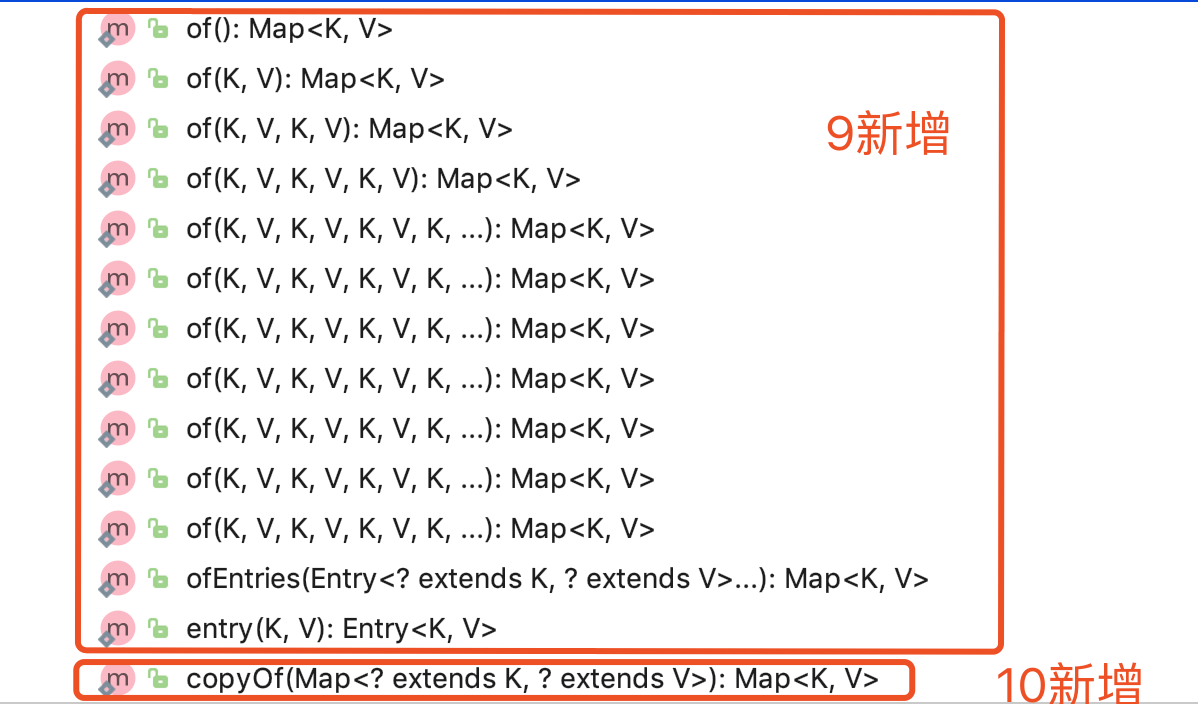# HashMap 源码阅读## Map 接口• 这些方法都是包私有的static方法；
• of()方法分别返回包含 0 - 9 个键值对的不可修改的Map；
• ofEntries()方法返回包含从给定的entries总提取出来的键值对的不可修改的* Map（不会包含给定的entries）；
• entry()方法返回包含键值对的不可修改的 Entry，不允许 null 作为 key 或 value；
• copyOf()返回一个不可修改的，包含给定 Map 的 entries 的 Map ，调用了ofEntries()方法.

## 数据结构

HashMap 是如何存储键值对的呢?

HashMap 有一个属性 table:

transient Node<K,V>[] table;


table 是一个 Node 的数组, 在首次使用和需要 resize 时进行初始化; 这个数组的长度始终是2的幂, 初始化时是0, 因此能够使用位运算来代替模运算.

HashMap的实现是装箱的(binned, bucketed), 一个 bucket 是 table 数组中的一个元素, 而 bucket 中的元素称为 bin .

static class Node<K,V> implements Map.Entry<K,V> {
final int hash;
final K key;
V value;
Node<K,V> next;

...
}


// 转换成红黑树
if (binCount >= TREEIFY_THRESHOLD - 1) // -1 for 1st
treeifyBin(tab, hash);

// 转换成链表结构
if (lc <= UNTREEIFY_THRESHOLD)


// table 为 null 或者 capacity 小于 MIN_TREEIFY_CAPACITY 会执行 resize() 而不是转换成树结构
if (tab == null || (n = tab.length) < MIN_TREEIFY_CAPACITY)
resize();


TreeNode 的结构和 TreeMap 相似, 并且实现了 tree 版本的一些方法:

static final class TreeNode<K,V> extends LinkedHashMap.Entry<K,V> {
TreeNode<K,V> parent;  // red-black tree links
TreeNode<K,V> left;
TreeNode<K,V> right;
TreeNode<K,V> prev;    // needed to unlink next upon deletion
boolean red;

...
}


• capacity，HashMap 的哈希表中桶的数量；
• initial capacity ，哈希表创建时桶的数量；
• load factor ，在 capacity 自动增加(resize())之前，哈希表允许的填满程度；
• threshold，下一次执行resize()时 size 的值 (capacity * load factor), 如果表没有初始化, 存放的是表的长度, 为0时表的长度将会是 DEFAULT_INITIAL_CAPACITY

static final int tableSizeFor(int cap) {
int n = -1 >>> Integer.numberOfLeadingZeros(cap - 1);
return (n < 0) ? 1 : (n >= MAXIMUM_CAPACITY) ? MAXIMUM_CAPACITY : n + 1;
}


static final float DEFAULT_LOAD_FACTOR = 0.75f;


initialCapacity 的默认值是16：

static final int DEFAULT_INITIAL_CAPACITY = 1 << 4; // aka 16


capacity 的最大值是1073741824：

static final int MAXIMUM_CAPACITY = 1 << 30;


float ft = ((float)s / loadFactor) + 1.0F;
int t = ((ft < (float)MAXIMUM_CAPACITY) ?
(int)ft : MAXIMUM_CAPACITY);
if (t > threshold)
threshold = tableSizeFor(t);


## hash() 方法

hash() 是 HashMap 用来计算 key 的 hash 值的方法, 这个方法并不是直接返回 key 的 hashCode() 方法的返回值, 而是将 hashCode 的高位移到低位后 再与原值异或.

static final int hash(Object key) {
int h;
return (key == null) ? 0 : (h = key.hashCode()) ^ (h >>> 16);
}


## resize()

resize() 方法负责初始化或扩容 table. 如果 table 为 null 初始化 table 为 一个长度为 threshold 或 DEFAULT_INITIAL_CAPACITY的表; 否则将 table 的长度加倍, 旧 table 中的元素要么呆在原来的 index 要么以2的幂为偏移量在新 table中移动:

final Node<K,V>[] resize() {
Node<K,V>[] oldTab = table;
int oldCap = (oldTab == null) ? 0 : oldTab.length;
int oldThr = threshold;
int newCap, newThr = 0;
if (oldCap > 0) {
if (oldCap >= MAXIMUM_CAPACITY) {
// 旧 table 的容量已经达到最大, 不扩容, 返回旧表
threshold = Integer.MAX_VALUE;
return oldTab;
}
else if ((newCap = oldCap << 1) < MAXIMUM_CAPACITY &&
oldCap >= DEFAULT_INITIAL_CAPACITY)
// 将旧容量加倍作为新表容量, 如果新表容量没达到容量最大值, 并且旧容量大于等于默认容量, threshold 加倍
newThr = oldThr << 1; // double threshold
}
else if (oldThr > 0) // initial capacity was placed in threshold
// 旧的threshold 不为 0 , 旧 threshold 作为新表的容量
newCap = oldThr;
else {               // zero initial threshold signifies using defaults
// 旧 threshold 为 0 , 用 DEFAULT_INITIAL_CAPACITY 作为新容量, 用默认值计算新 threshold
newCap = DEFAULT_INITIAL_CAPACITY;
}
if (newThr == 0) {
// 之前没有计算过新 threshold , 计算 threshold
float ft = (float)newCap * loadFactor;
newThr = (newCap < MAXIMUM_CAPACITY && ft < (float)MAXIMUM_CAPACITY ?
(int)ft : Integer.MAX_VALUE);
}
threshold = newThr;
@SuppressWarnings({"rawtypes","unchecked"})
// 创建新表数组, 更新表引用
Node<K,V>[] newTab = (Node<K,V>[])new Node[newCap];
table = newTab;
if (oldTab != null) {
// 将旧表中的元素移动到新表
for (int j = 0; j < oldCap; ++j) {
// 遍历旧表
Node<K,V> e;
if ((e = oldTab[j]) != null) {
// 帮助 GC
oldTab[j] = null;
if (e.next == null)
// 这个桶里只有一个元素, 此处用位运算代替了模运算
newTab[e.hash & (newCap - 1)] = e;
else if (e instanceof TreeNode)
// 如果这个 bucket 的结构是树, 将这个 bucket 中的元素分为高低两部分((e.hash & bit) == 0 就分在低的部分, bit 是 oldCap), 低的部分留在原位, 高的部分放到 newTab[j + oldCap]; 如果某一部分的元素个数小于 UNTREEIFY_THRESHOLD 将这一部分转换成链表形式, 否则就形成新的树结构
((TreeNode<K,V>)e).split(this, newTab, j, oldCap);
else { // preserve order
// 将普通结构的 bucket 中的元素分为高低两部分, 低的部分留在原位, 高的部分放到 newTab[j + oldCap]
Node<K,V> loHead = null, loTail = null;
Node<K,V> hiHead = null, hiTail = null;
Node<K,V> next;
do {
next = e.next;
if ((e.hash & oldCap) == 0) {
if (loTail == null)
else
loTail.next = e;
loTail = e;
}
else {
if (hiTail == null)
else
hiTail.next = e;
hiTail = e;
}
} while ((e = next) != null);
if (loTail != null) {
loTail.next = null;
}
if (hiTail != null) {
hiTail.next = null;
}
}
}
}
}
return newTab;
}


• 扩容前 table.length 是 0000 1000 记为 oldCap , table.length - 1 是 0000 0111 记为 oldN;
• 扩容后 table.length 是 0001 0000 记为 newCap, table.length - 1 为 0000 1111 记为 newN;
• 有两个Node, hash ( hash() 方法得到的值)分别为 0000 1101 和 0000 0101 记为 n1 和 n2;

## 查找

public V get(Object key) {
Node<K,V> e;
return (e = getNode(hash(key), key)) == null ? null : e.value;
}

@Override
public V getOrDefault(Object key, V defaultValue) {
Node<K,V> e;
return (e = getNode(hash(key), key)) == null ? defaultValue : e.value;
}


### getNode() 方法

final Node<K,V> getNode(int hash, Object key) {
Node<K,V>[] tab; Node<K,V> first, e; int n; K k;
if ((tab = table) != null && (n = tab.length) > 0 &&
(first = tab[(n - 1) & hash]) != null) {
// table 已经被初始化且 table 的长度不为 0 且 对应的 bucket 里有 bin
if (first.hash == hash && // always check first node
((k = first.key) == key || (key != null && key.equals(k))))
// 第一个节点的 key 和 给定的 key 相同
return first;
if ((e = first.next) != null) {
// bucket 中还有下一个 bin
if (first instanceof TreeNode)
// 是树结构的 bucket, 调用树版本的 getNode 方法
return ((TreeNode<K,V>)first).getTreeNode(hash, key);
do {
// 在普通的链表中查找 key
if (e.hash == hash &&
((k = e.key) == key || (key != null && key.equals(k))))
return e;
} while ((e = e.next) != null);
}
}
return null;
}


## 遍历

### fast-fail 和 modCount

HashMap 不是线程安全的, 并且实现了 fast-fail 机制. 当一个迭代器被创建的时候(或者迭代器自身的 remove() 方法被调用), 会记录当前的 modCount 作为期待中的 modCount, 并在操作中先检查当前 modCount 是不是和旧的 modCount 相同, 不同则会抛出ConcurrentModificationException.

## 新增和更新

1.8 之前有4个方法和构造器能够往 HashMap 中添加键值对: 以一个Map为参数的构造器、put()putAll()putIfAbsent(),

public HashMap(Map<? extends K, ? extends V> m) {
putMapEntries(m, false);
}

public V put(K key, V value) {
return putVal(hash(key), key, value, false, true);
}

public void putAll(Map<? extends K, ? extends V> m) {
putMapEntries(m, true);
}

@Override
public V putIfAbsent(K key, V value) {
return putVal(hash(key), key, value, true, true);
}


### putVal() 方法

final V putVal(int hash, K key, V value, boolean onlyIfAbsent,
boolean evict) {
Node<K,V>[] tab; Node<K,V> p; int n, i;
if ((tab = table) == null || (n = tab.length) == 0)
// table 未被初始化或者长度为 0 时, 执行 resize()
n = (tab = resize()).length;
if ((p = tab[i = (n - 1) & hash]) == null)
// 对应的 bucket 里没有元素, 新建一个普通 Node 放到这个位置
tab[i] = newNode(hash, key, value, null);
else {
Node<K,V> e; K k;
if (p.hash == hash &&
((k = p.key) == key || (key != null && key.equals(k))))
// 第一个节点的 key 和 给定的 key 相同
e = p;
else if (p instanceof TreeNode)
// 树结构, 调用树版本的 putVal, 如果树结构中存在 key, 将会返回相应的 TreeNode, 否则返回 null
e = ((TreeNode<K,V>)p).putTreeVal(this, tab, hash, key, value);
else {
for (int binCount = 0; ; ++binCount) {
if ((e = p.next) == null) {
// 在链表中没有找到 key, 新建一个节点放到链表末尾
p.next = newNode(hash, key, value, null);
if (binCount >= TREEIFY_THRESHOLD - 1) // -1 for 1st
// 当前桶转换成树结构
treeifyBin(tab, hash);
break;
}
if (e.hash == hash &&
((k = e.key) == key || (key != null && key.equals(k))))
// key 相同 break
break;
p = e;
}
}
if (e != null) { // existing mapping for key
// key 在 map 中存在
V oldValue = e.value;
if (!onlyIfAbsent || oldValue == null)
// 覆盖旧值
e.value = value;
afterNodeAccess(e);
return oldValue;
}
}
// key 之前在 map 中不存在, 发生了结构变化, modCount 增加 1
++modCount;
if (++size > threshold)
// 扩容
resize();
afterNodeInsertion(evict);
return null;
}


HashMap 提供了三个回调方法:

void afterNodeAccess(Node<K,V> p) { }
void afterNodeInsertion(boolean evict) { }
void afterNodeRemoval(Node<K,V> p) { }


### putMapEntries() 方法

putMapEntries()方法就简单多了

final void putMapEntries(Map<? extends K, ? extends V> m, boolean evict) {
int s = m.size();
if (s > 0) {
if (table == null) { // pre-size
// table 还没有初始化, 计算出 threshold
float ft = ((float)s / loadFactor) + 1.0F;
int t = ((ft < (float)MAXIMUM_CAPACITY) ?
(int)ft : MAXIMUM_CAPACITY);
if (t > threshold)
threshold = tableSizeFor(t);
}
else if (s > threshold)
// s 超过了 threshold, 扩容
resize();
for (Map.Entry<? extends K, ? extends V> e : m.entrySet()) {
// 调用 putVal() 方法, 将键值对放进 map
K key = e.getKey();
V value = e.getValue();
putVal(hash(key), key, value, false, evict);
}
}
}


## 删除

public V remove(Object key) {
Node<K,V> e;
return (e = removeNode(hash(key), key, null, false, true)) == null ?
null : e.value;
}

@Override
public boolean remove(Object key, Object value) {
return removeNode(hash(key), key, value, true, true) != null;
}

public void clear() {
Node<K,V>[] tab;
modCount++;
if ((tab = table) != null && size > 0) {
size = 0;
for (int i = 0; i < tab.length; ++i)
tab[i] = null;
}
}


### removeNode() 方法

removeNode() 方法有5个参数, 说明一下其中两个:

• matchValue 为 true 时, 只在 value 符合的情况下删除;
• movable 为 false 时, 删除时不移动其他节点, 只给树版本的删除使用.
final Node<K,V> removeNode(int hash, Object key, Object value,
boolean matchValue, boolean movable) {
Node<K,V>[] tab; Node<K,V> p; int n, index;
if ((tab = table) != null && (n = tab.length) > 0 &&
(p = tab[index = (n - 1) & hash]) != null) {
// table 已经被初始化且 table 的长度不为 0 且 对应的 bucket 里有 bin
Node<K,V> node = null, e; K k; V v;
if (p.hash == hash &&
((k = p.key) == key || (key != null && key.equals(k))))
// 第一个的 key 和给定的 key 相同
node = p;
else if ((e = p.next) != null) {
// bucket 中有不止一个 bin
if (p instanceof TreeNode)
// 树结构, 调用树版本的 getNode
node = ((TreeNode<K,V>)p).getTreeNode(hash, key);
else {
// 在普通的 bucket 中查找 node
do {
if (e.hash == hash &&
((k = e.key) == key ||
(key != null && key.equals(k)))) {
node = e;
break;
}
p = e;
} while ((e = e.next) != null);
}
}
if (node != null && (!matchValue || (v = node.value) == value ||
(value != null && value.equals(v)))) {
// 找到了 node , 并且符合删除条件
if (node instanceof TreeNode)
// 树结构, 调用树版本的 removeNode , 如果节点过少, 会转换成链表结构
((TreeNode<K,V>)node).removeTreeNode(this, tab, movable);
else if (node == p)
// node 是链表的第一个元素
tab[index] = node.next;
else
// 不是第一个元素
p.next = node.next;
// 结构变化 modCount + 1
++modCount;
--size;
afterNodeRemoval(node);
return node;
}
}
return null;
}


## 总结

• HashMap 是一个基于哈希表的装箱了的 Map 的实现; 它的数据结构是一个桶的数组, 桶的结构可能是单向链表或者红黑树, 大部分是链表.
• table 的容量是2的幂, 因此可以用更高效的位运算替代模运算.
• HashMap 使用的 hash 值, 并不是 key 的 hashCode()方法所返回的值, 详细还是看上面吧.
• 一个普通桶中的 bin 的数量超过 TREEIFY_THRESHOLD, 并且 table 的容量大于 MIN_TREEIFY_CAPACITY, 这个桶会被转换成树结构; 如果 bin 数量大于TREEIFY_THRESHOLD , 但 table 容量小于 MIN_TREEIFY_CAPACITY, 会进行扩容.
• 每次扩容新 table 的容量是老 table 的 2 倍.
• 扩容时, 会将原来下标为 index 的桶里的 bin 分为高低两个部分, 高的部分放到 newTab[index + oldCap] 上, 低的部分放在原位; 如果某部分的 bin 的个数小于 UNTREEIFY_THRESHOLD 树结构将会转换成链表结构.
• ... ... (不想写了, 以后再改吧)
posted @ 2019-01-06 04:26  -.-|  阅读(198)  评论(0编辑  收藏